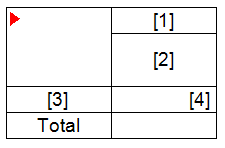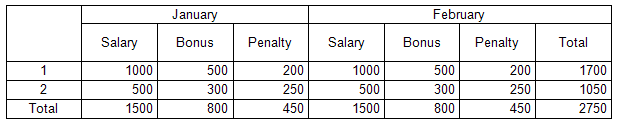• Home
• /
• White Papers
• /
• How to create your own total in the matrix FastReport.Net

# How to create your own total in the matrix FastReport.NetDmitriy Fedyashov

The Matrix object in FastReport .Net performs typical tasks for displaying summary tables very well. But, when the tasks are not standard, only the report script will help us. It is in the script itself you can implement almost any requirement.

As you know, the matrix has a built-in function to display totals by fields and columns. Usually, the total in the summary table is the amount. However, what if you want a total with your own calculation formula. And if you want to display totals selectively for certain columns?

To do all this you need to disable the standard totals and create your own column, in which your total will be calculated. But, if you still use the standard totals, then you need to use the AfterTotals matrix event to make their values also appear in the user total. It will happen after the construction of the matrix with all the standard results, so that all the data will be at our disposal. This event does not allow you to add and modify data to matrixes, that is, use the AddValue and SetValue methods.

So, let's have a look at an example.

Create a matrix with a simple template:To create a dimension or metric in a matrix, you need to insert an expression into the corresponding cell. Because we fill the matrix from the code, then we need dummy expressions just to create the structure. To do this, place any expression from the "Data" panel in the cell and clean the text of the expression using the expression editor.

Create the AfterData event handler for the matrix. In it, we will add data:

 ```1 2 3 4 5 6 7 8 9 10 11 12 13 14 15 16 17 ``` ```private void Matrix1_AfterData(object sender, EventArgs e) { Matrix1.AddValue(new Object[] { "January", "Salary" }, new Object[] { "1" }, new Object[] { 1000}); Matrix1.AddValue(new Object[] { "January", "Bonus" }, new Object[] { "1" }, new Object[] { 500}); Matrix1.AddValue(new Object[] { "January", "Penalty" }, new Object[] { "1" }, new Object[] { 200}); Matrix1.AddValue(new Object[] { "February", "Salary" }, new Object[] { "1" }, new Object[] { 1000}); Matrix1.AddValue(new Object[] { "February", "Bonus" }, new Object[] { "1" }, new Object[] { 500}); Matrix1.AddValue(new Object[] { "February", "Penalty" }, new Object[] { "1" }, new Object[] { 200}); Matrix1.AddValue(new Object[] { "February", "Total" }, new Object[] { "1" }, new Object[] { 0});   Matrix1.AddValue(new Object[] { "January", "Salary" }, new Object[] { "2" }, new Object[] { 500}); Matrix1.AddValue(new Object[] { "January", "Bonus" }, new Object[] { "2" }, new Object[] { 300}); Matrix1.AddValue(new Object[] { "January", "Penalty" }, new Object[] { "2" }, new Object[] { 250}); Matrix1.AddValue(new Object[] { "February", "Salary" }, new Object[] { "2" }, new Object[] { 500}); Matrix1.AddValue(new Object[] { "February", "Bonus" }, new Object[] { "2" }, new Object[] { 300}); Matrix1.AddValue(new Object[] { "February", "Penalty" }, new Object[] { "2" }, new Object[] { 250}); Matrix1.AddValue(new Object[] { "February", "Total" }, new Object[] { "2" }, new Object[] { 0});}```

Here, we add values for each cell in the matrix. In our case, these are two rows of data. Please note that we added only one total for February. While it has a zero value, but soon we will calculate it in the AfterTotals event.

Next, we need a method for obtaining the value of a cell:

 ```1 2 3 4 5 ``` ``` private float GetValue(int columnIndex) { object value = Matrix1.Data.GetValue(columnIndex, rowIndex, 0); return new Variant(value); }```

It's simple - we pass the column index, and we get the value.

Also we need a class variable - rowIndex:

private int rowIndex;

Now let's add a method of assigning a value to a cell:

 ```1 2 3 4 5 ``` ```private void SetValue(string complexValue, float value) { int columnIndex = Matrix1.Data.Columns.Find(complexValue.Split(';')); Matrix1.Data.SetValue(columnIndex, rowIndex, value); }```

Now, let's move on to calculating the result. Add the AfterTotals event handler for the matrix:

 ```1 2 3 4 5 6 7 8 9 10 11 12 13 14 15 16 ``` ```private void Matrix1_AfterTotals(object sender, EventArgs e) { int[] rowIndices = Matrix1.Data.Rows.GetTerminalIndices(); for (int i = 0; i < rowIndices.Length; i++) { rowIndex = rowIndices[i];   int[] columnIndices = Matrix1.Data.Columns.GetTerminalIndices(new Object[] { "February" }); float oplataSum = 0; foreach (int columnIndex in columnIndices) { oplataSum += GetValue(columnIndex); } SetValue("February;Total", oplataSum); } }```

Here, we get an array of indexes of matrix rows. Then, we cycle through these row indices. For each row, we get an array of column indices. In the cycle for the column indexes, we generate an increasing amount. At the end, we assign the received amount to the "February; Total" cell.

Now, run the report:The result, as expected, is output for the group February. And the amounts from the "staff" total are also processed.

In this way, we can create our own totals for the desired columns and rows.

Similar articles:

back